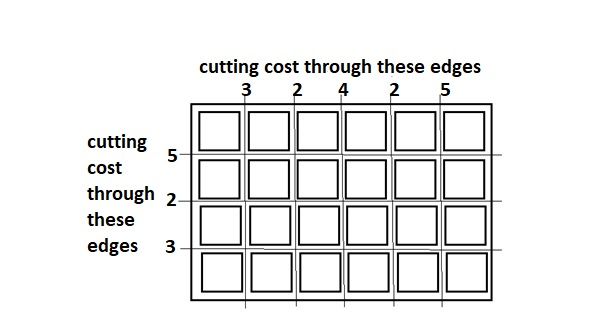# Minimum Cost to cut a board into squares in C++

## Concept

Suppose a board of length p and width q is given, we require to break this board into p*q squares such that cost of breaking is least. For this board,cutting cost for each edge will be given. In a nutshell, we require selecting such a sequence of cutting such that cost is minimized.

ExamplesWith respect of above board optimal way to cut into square is −

Total minimum cost in above case is 65. It is computed evaluated implementing following steps.

Initial Value : Total_cost = 0
Total_cost = Total_cost + edge_cost * total_pieces
Cost 5 Horizontal cut Cost = 0 + 5*1 = 5
Cost 5 Vertical cut Cost = 5 + 5*2 = 15
Cost 4 Vertical cut Cost = 15 + 4*2 = 23
Cost 3 Horizontal cut Cost = 23 + 3*3 = 32
Cost 3 Vertical cut Cost = 32 + 3*3 = 41
Cost 2 Horizontal cut Cost = 41 + 2*4 = 49
Cost 2 Vertical cut Cost = 49 + 2*4 = 57
Cost 2 Vertical cut Cost = 57 + 2*4 = 65

## Method

This type of problem can be solved implementing greedy approach. If total cost is treated by S, then S = b1x1 + b2x2 … + bkxk, where xi is treated as a cost of certain edge cutting and bi is corresponding coefficient, The coefficient bi is determined by thetotal number of cuts we have competed implementing edge xi at the end of the cutting process.

We should notice that sum of the coefficients are always constant, hence we want to compute a distribution of bi obtainable such that S is least. To do so we accomplish cuts on largest cost edge as soon as possible, which will reach to optimal S. If we encounter several edges having the equal cost, we can remove or cut any one of them first.

## C++ Program

Following is the solution implementing above approach, first we sorted the edge cutting costs in reverse order, and then we loop in them from higher cost to lower cost building our solution. Each time we select an edge, counterpart count is incremented by 1, which is to be multiplied each time with corresponding edge cutting cost.

## Example

Live Demo

// C++ program to divide a board into p*q squares
#include <bits/stdc++.h>
using namespace std;
int minimumCostOfBreaking(int X1[], int Y1[], int p, int q){
int res1 = 0;
sort(X1, X1 + p, greater<int>());
sort(Y1, Y1 + q, greater<int>());
int hzntl = 1, vert = 1;
int i = 0, j = 0;
while (i < p && j < q){
if (X1[i] > Y1[j]){
res1 += X1[i] * vert;
hzntl++;
i++;
}
else{
res1 += Y1[j] * hzntl;
vert++;
j++;
}
}
int total = 0;
while (i < p)
total += X1[i++];
res1 += total * vert;
total = 0;
while (j < q)
total += Y1[j++];
res1 += total * hzntl;
return res1;
}
int main(){
int p = 6, q = 4;
int X1[p-1] = {3, 2, 4, 2, 5};
int Y1[q-1] = {5, 2, 3};
cout << minimumCostOfBreaking(X1, Y1, p-1, q-1);
return 0;
}

## Output

65